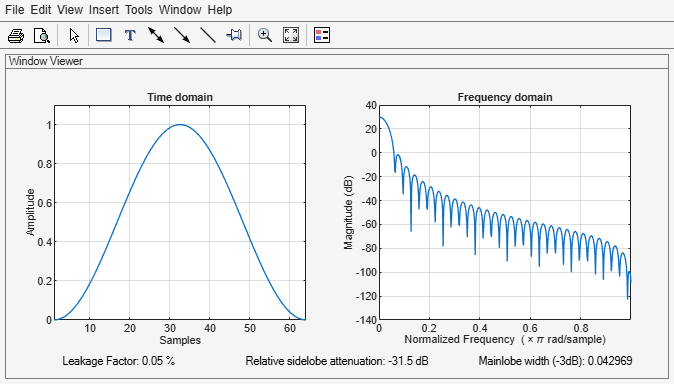# hann

Hann (Hanning) window

## Syntax

``w = hann(L)``
``w = hann(L,sflag)``

## Description

example

````w = hann(L)` returns an `L`-point symmetric Hann window.```
````w = hann(L,sflag)` returns a Hann window using the window sampling specified by `sflag`.```

## Examples

collapse all

Create a 64-point Hann window. Display the result using `wvtool`.

```L = 64; wvtool(hann(L))```## Input Arguments

collapse all

Window length, specified as a positive integer.

Data Types: `single` | `double`

Window sampling, specified as one of the following:

• `'symmetric'` — Use this option when using windows for filter design.

• `'periodic'` — This option is useful for spectral analysis because it enables a windowed signal to have the perfect periodic extension implicit in the discrete Fourier transform. When `'periodic'` is specified, `hann` computes a window of length L + 1 and returns the first L points.

## Output Arguments

collapse all

Hann window, returned as a column vector.

## Algorithms

The following equation generates the coefficients of a Hann window:

`$w\left(n\right)=\begin{array}{cc}0.5\left(1-\mathrm{cos}\left(2\pi \frac{n}{N}\right)\right),& 0\le n\le N\end{array}.$`

The window length L = N + 1.

 Oppenheim, Alan V., Ronald W. Schafer, and John R. Buck. Discrete-Time Signal Processing. Upper Saddle River, NJ: Prentice Hall, 1999.